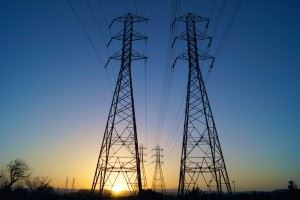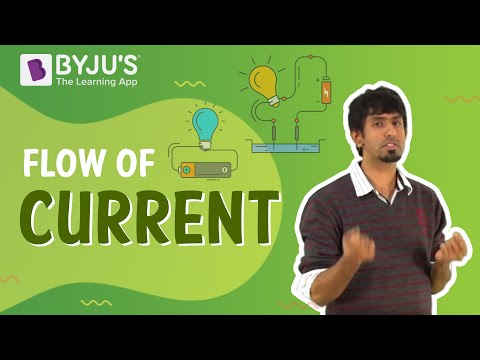# Electrical Energy and Power

Electrical energy is an important concept in science, yet it is frequently misunderstood. So what exactly is electrical energy and what are some of the rules applied when it is used in calculations? In this article, let us find answers to these questions.

## What is an Electrical Energy?

Electrical energy is the energy derived from electric potential energy or kinetic energy of the charged particles. In general, it is referred to as the energy that has been converted from electric potential energy. We can define electrical energy as the energy generated by the movement of electrons from one point to another. The movement of charged particles along/through a medium (say wire) constitute current or electricity.

### Electrical Energy FormulaA cell has two terminals – a negative and a positive terminal. The negative terminal has the excess of electrons whereas the positive terminal has a deficiency of electrons. Let us take the positive terminal as A and the electrical potential at A is given by V(A). Similarly, the negative terminal is B and the electrical potential at B is given by V(B). Electric current flows from A to B, and thus V(A) > V (B).

The potential difference between A and B is given by

V = V(A) – V(B) > 0

Mathematically, electric current is defined as the rate of flow of charge through the cross-section of a conductor.

Thus, it is given by I = ∆Q/ ∆t where I is the electric current and ∆Q is the quantity of electric charge flowing through a point in time ∆t.

The potential energy of charge Q at A is Q V(A) and at B, it is Q V(B). So the change in the potential energy is given by

∆Upot = Final potential energy – Initial potential energy

= ∆Q [(V (B) – V (A)] = –∆Q V

= –I V∆t (Since I = ∆Q/ ∆t)

If we take the kinetic energy of the system into account, it would also change if the charges inside the conductor moved without collision. This is to keep the total energy of the system unchanged. Thus, by conservation of total energy, we have:

∆K = –∆Upot

Or ∆K = I V∆t > 0

Thus, in the electric field, if the charges move freely across the conductor, there would be an increase in the kinetic energy as they move.

When the charges collide, the energy gained by them is shared between the atoms. Consequently, the vibration of the atoms increases resulting in the heating up of the conductor. Thus, some amount of energy is dissipated in the form of heat in an actual conductor.

### Units of Electrical Energy

The basic unit of electrical energy is the joule or watt-second. An electrical energy is said to be one joule when one ampere of current flows through the circuit for a second when the potential difference of one volt is applied across it. The commercial unit of electrical energy is the kilowatt-hour (kWh) which is also knwon as the Board of trade unit (B.O.T).
1 kwh = 1000 × 60 × 60 watt – second
1 kwh = 36 × 105 Ws or Joules
Generally, one kwh is called one unit.

### What are some examples of electrical energy?

A few examples of electrical energy are:

• In a car battery, a chemical reaction results in the formation of an electron which possesses the energy to move in an electric current. These moving charges provide electrical energy to the circuits in the car.
• Lightning, during a thunderstorm, is an example of electrical energy – what we see as lightning is nothing but electricity discharging in the atmosphere.
• Electric eels generate electrical energy and use it against predators for defence.

### Electrical Energy Into Mechanical Energy

Electrical energy can be converted into other forms of energy like heat energy, light energy, motion etc. The best-known example are:

• Fan: The motor in Fan converts electrical energy into mechanical energy
• Bulb: Here the electrical energy is converted into light energy.

## Summary

Some of the keynotes on electrical energy is tabulated below

 Definition Electrical energy can be due to either kinetic energy or potential energy. Mostly it is due to potential energy, which is energy stored due to the relative positions of charged particles or electric fields. Symbol E Units Joule (J) Kilowatt-hour(kWh) Electron-Volt(eV) Formula E = QVWhere, Q is charge V is the potential difference Examples Electrical charges moving through a wire or electricity. Lightning. Batteries. Static electricity. Electric wheels generate electrical energy. Electricity stored in capacitors. Audio speakers. Doorbells. Uses Lighting, cooling, heating. Operating appliances, electronics, computers, machinery. Public transportation systems. Facts Electricity travels at the speed of light that is more than 186,000 miles per second. A spark of static electricity can measure up to 3,000 volts. A bolt of lightning can measure up to 3,000,000 volts and it lasts less than one second.

## What is Electric Power?

Electric power Definition – It is the rate at which work is done or energy is transformed in an electrical circuit. Simply put, it is a measure of how much energy is used in a span of time.

In physics, the rate of transfer of electrical energy by an electrical circuit per unit time is called electrical power. Here electrical energy can be either kinetic energy or potential energy. In most of the cases, potential energy is considered, which is the energy stored due to the relative positions of charged particles or electric fields. Electrical power is denoted by P and measured using Watt.

 Symbol P SI Unit Watt, joule per second Scalar or Vector Scalar Quantity Formula P=VI Where, V is the potential difference (volts) I is the electric current

We talked about the energy that is dissipated due to the heating up of the conductor. The energy dissipated in time interval ∆t is given by

∆W = I V∆t

And the energy dissipated per unit time is actually the power dissipated, which is given by P = ∆W/∆t. But we know the formula for power is given by P = I V

Hence, according to Ohm’s law, V = IR. Substituting we have,

P = I2R

Or

P = V2/R

It is this power which is responsible for heating up the coil of a bulb, which gives out heat and light.

## Practice Questions On Electric Energy And Power

Q1: Define electrical energy.

Ans: Electrical energy can be due to either kinetic energy or potential energy. Mostly it is due to potential energy, which is energy stored due to the relative positions of charged particles or electric fields.

Q2: Write the units fo electrical energy.

Ans:  The units of electrical energy are joules, kilowatt hour, electron-volt

Q3: Write the uses of electrical energy.

Ans:  The uses of electrical energy are plenty. Some of them are Lighting, cooling, heating. Operating appliances, electronics, computers, machinery. Public transportation systems

Q4: What is the speed of electricity?

Ans: Electricity travels at the speed of light that is more than 186,000 miles per second.

Q5: What constitutes electricity?

Ans:  The movement of mobile electrons constitute electricity.

Q6: Define electric Power

Ans:electric power measure of the rate of electrical energy transfer by an electric circuit per unit time.

Q7: Write the SI unit of electric power

Ans: The SI unit of electric power is watt

Q8: Write the electric power formula and explain the terms

Ans: The electric power is given by P = VI.

Where V is the potential difference, I is the electric current and P is the electric power.

Q9: Is electric power a scalar quantity or vector quantity?

Ans: Electric power is the scalar quantity

Q10: How can we express power using Ohms law?

Ans: Power formula can be rewritten using Ohms law as P =I2R or P = V2/R.

Where V is the potential difference, I is the electric current, R is the resistance and P is the electric power

Hope you have understood about Electric energy and power, How it is defined, its Symbol, Units, Formula, Terms. For a better understanding of current electricity do read the related articles and answer the practice questions.

Related Physics Concepts:

Stay tuned with BYJU’S for more such interesting articles. Also, register to “BYJU’S-The Learning App” for loads of interactive, engaging physics related videos and an unlimited academic assist.

## Why shouldn’t you touch electrical equipment with wet hands? Find out answer to this question by watching the video.Test Your Knowledge On Electrical Energy And Power!

#### 1 Comment

1. Rajdeep Sarkar